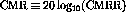Next: Current Limiting and Slew Up: Analysis Using Finite Open-Loop Previous: Input Impedance

## Voltage and Current Offsets

Since op-amps are generally DC coupled, there will appear a nonzero output even when the inputs are grounded or connected to give no input signal. The voltage offset is the result of slightly different transistors making up the differential input stage. The voltage offset can be reduced by using an externally-adjustable bias resistor (voltage offset null circuitry).

The current offsets at the inverting and non-inverting input terminals are usually base currents into two identical bipolar transistors. Thus their difference can be expected to be much less than either base current alone. Using this fact the student should be able to explain the reason for having an extra resistor between the non-inverting input and ground for the inverting amplifier. The resistor should have a value equal to the input resistor and feedback resistor in parallel.

We define the following:

output offset voltage - The voltage at the output when the input voltage is zero (input terminals grounded).
common mode voltage - The voltage at the output when the voltage at the inverting and non-inverting inputs are equal.
common mode rejection ratio (CMRR) - The ratio of the op-amp gain when operating in differential mode to the gain when operating in common mode.
common mode rejection (CMR) - The ability to respond to only differences at the input terminals:.

Doug Gingrich
Tue Jul 13 16:55:15 EDT 1999